Hostname: page-component-797576ffbb-58z7q Total loading time: 0 Render date: 2023-12-05T22:20:37.975Z Has data issue: false Feature Flags: { "corePageComponentGetUserInfoFromSharedSession": true, "coreDisableEcommerce": false, "useRatesEcommerce": true } hasContentIssue false

# HOLOMORPHIC FUNCTIONS WITH IMAGE OF GIVEN LOGARITHMIC OR ELLIPTIC CAPACITY

Published online by Cambridge University Press:  08 March 2013

## Abstract

Core share and HTML view are not possible as this article does not have html content. However, as you have access to this content, a full PDF is available via the ‘Save PDF’ action button.

For holomorphic functions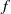$f$ in the unit disk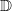$\mathbb{D}$ with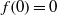$f(0)= 0$, we prove a modulus growth bound involving the logarithmic capacity (transfinite diameter) of the image. We show that the pertinent extremal functions map the unit disk conformally onto the interior of an ellipse. We prove a modulus growth bound for elliptically schlicht functions in terms of the elliptic capacity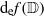${\mathrm{d} }_{\mathrm{e} } f( \mathbb{D} )$ of the image. We also show that the function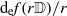${\mathrm{d} }_{\mathrm{e} } f(r \mathbb{D} )/ r$ is increasing for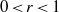$0\lt r\lt 1$.

Type
Research Article
Information

## References

Anderson, G. D., Vamanamurthy, M. K. and Vuorinen, M., Conformal Invariants, Inequalities, and Quasiconformal Maps (Wiley, New York, 1997).Google Scholar
Betsakos, D., ‘Elliptic, hyperbolic, and condenser capacity; geometric estimates for elliptic capacity’, J. Anal. Math. 96 (2005), 3755.Google Scholar
Burckel, R. B., Marshall, D. E., Minda, D., Poggi-Corradini, P. and Ransford, T. J., ‘Area, capacity and diameter versions of Schwarz’s lemma’, Conform. Geom. Dyn. 12 (2008), 133152.Google Scholar
Cunningham, R., ‘Univalent functions of given transfinite diameter: a maximum modulus problem’, Ann. Acad. Sci. Fenn. Math. 18 (1993), 249271.Google Scholar
Dubinin, V. N., ‘Symmetrization in the geometric theory of functions of a complex variable’, Uspekhi Mat. Nauk 49 (1994), 376 (in Russian); translation in Russian Math. Surveys 49 (1994), 1–79.Google Scholar
Duren, P. and Kühnau, R., ‘Elliptic capacity and its distortion under conformal mapping’, J. Anal. Math. 89 (2003), 317335.Google Scholar
Garnett, J. B. and Marshall, D. E., Harmonic Measure (Cambridge University Press, Cambridge, 2005).Google Scholar
Hayman, W. K., Multivalent Functions, 2nd edn (Cambridge University Press, Cambridge, 1994).Google Scholar
Jenkins, J. A., Univalent Functions and Conformal Mapping (Springer, Berlin, 1958).Google Scholar
Kraus, D. and Roth, O., ‘Weighted distortion in conformal mapping in Euclidean, hyperbolic and elliptic geometry’, Ann. Acad. Sci. Fenn. Math. 31 (2006), 111130.Google Scholar
Kühnau, R., ‘Über vier Klassen schlichter Funkionen’, Math. Nachr. 50 (1971), 1726.Google Scholar
Kühnau, R., ‘Variation of diametrically symmetric or elliptically schlicht conformal mappings’, J. Anal. Math. 89 (2003), 303316.Google Scholar
Pouliasis, S., ‘Condenser capacity and meromorphic functions’, Comput. Methods Funct. Theory 11 (2011), 237245.Google Scholar
Ransford, T., Potential Theory in the Complex Plane (Cambridge University Press, Cambridge, 1995).Google Scholar
Remmert, R., Classical Topics in Complex Function Theory (Springer, New York, 1998).Google Scholar
Schippers, E. D., ‘Estimates on kernel functions of elliptically schlicht domains’, Comput. Methods Funct. Theory 2 (2002), 579596.Google Scholar
Shah, T.-S., ‘On the moduli of some classes of analytic functions’, Acta Math. Sinica 5 (1955), 439454 (in Chinese. English summary).Google Scholar